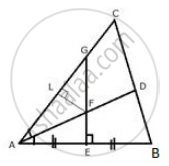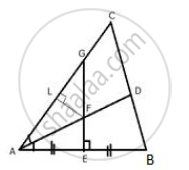# The Given Figure Shows a Triangle Abc in Which Ad Bisects Angle Bac. Eg is Perpendicular Bisector of Side Ab Which Intersects Ad at Point F. Prove That: - Mathematics

Sum

The given figure shows a triangle ABC in which AD bisects angle BAC. EG is perpendicular bisector of side AB which intersects AD at point F.
Prove that:(ii) F is equidistant from AB and AC.

#### SolutionConstruction: Draw LF ⊥ AC
Proof: In Δ AFL and ΔAFE,
∠FEA = ∠FLA (Each = 90°)
∠LAF = FAE (AD bi sects ∠BAC)
AF = AF (common)
∴ By angle – Angle side criterion of congruence,
ΔAFL ≅ AFE (AAS postulate)
The corresponding parts of the congruent triangles are congruent.
∴ FE = FL (CPCT)
Hence, F is equidistant from AB and AC.

Concept: Theorems Based on Loci
Is there an error in this question or solution?

#### APPEARS IN

Selina Concise Maths Class 10 ICSE
Chapter 16 Loci (Locus and Its Constructions)
Exercise 16 (A) | Q 11.2 | Page 238